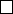5Eigenvalues and eigenvectors

IA Vectors and Matrices5.1 Preliminaries and definitions
Theorem
(Fundamental theorem of algebra)
.
Let
p
(
z
) be a polynomial of degree
m 1, i.e.
p(z) =
m
X
j=0
c
j
z
j
,
where c
j
C and c
m
6= 0.
Then
p
(
z
) = 0 has precisely
m
(not necessarily distinct) roots in the complex
plane, accounting for multiplicity.
Note that we have the disclaimer “accounting for multiplicity”. For example,
x
2
2
x
+ 1 = 0 has only one distinct root, 1, but we say that this root has
multiplicity 2, and is thus counted twice. Formally, multiplicity is defined as
follows:
Definition
(Multiplicity of root)
.
The root
z
=
ω
has multiplicity
k
if (
z ω
)
k
is a factor of p(z) but (z ω)
k+1
is not.
Example.
Let
p
(
z
) =
z
3
z
2
z
+ 1 = (
z
1)
2
(
z
+ 1). So
p
(
z
) = 0 has roots
1, 1, 1, where z = 1 has multiplicity 2.
Definition
(Eigenvector and eigenvalue)
.
Let
α
:
C
n
C
n
be a linear map
with associated matrix A. Then x 6= 0 is an eigenvector of A if
Ax = λx
for some
λ
.
λ
is the associated eigenvalue. This means that the direction of the
eigenvector is preserved by the mapping, but is scaled up by λ.
There is a rather easy way of finding eigenvalues:
Theorem. λ is an eigenvalue of A iff
det(A λI) = 0.
Proof.
(
) Suppose that
λ
is an eigenvalue and
x
is the associated eigenvector.
We can rearrange the equation in the definition above to
(A λI)x = 0
and thus
x ker(A λI)
But
x 6
=
0
. So
ker
(
AλI
) is non-trivial and
det
(
AλI
) = 0. The (
) direction
is similar.
Definition
(Characteristic equation of matrix)
.
The characteristic equation of
A is
det(A λI) = 0.
Definition
(Characteristic polynomial of matrix)
.
The characteristic polynomial
of A is
p
A
(λ) = det(A λI).
From the definition of the determinant,
p
A
(λ) = det(A λI)
= ε
j
1
j
2
···j
n
(A
j
1
1
λδ
j
1
1
) ···(A
j
n
n
λδ
j
n
n
)
= c
0
+ c
1
λ + ··· + c
n
λ
n
for some constants c
0
, ··· , c
n
. From this, we see that
(i) p
A
(
λ
) has degree
n
and has
n
roots. So an
n ×n
matrix has
n
eigenvalues
(accounting for multiplicity).
(ii)
If
A
is real, then all
c
i
R
. So eigenvalues are either real or come in
complex conjugate pairs.
(iii) c
n
= (
1)
n
and
c
n1
= (
1)
n1
(
A
11
+
A
22
+
···
+
A
nn
) = (
1)
n1
tr
(
A
).
But c
n1
is the sum of roots, i.e. c
n1
= (1)
n1
(λ
1
+ λ
2
+ ···λ
n
), so
tr(A) = λ
1
+ λ
2
+ ··· + λ
n
.
Finally,
c
0
=
p
A
(0) =
det
(
A
). Also
c
0
is the product of all roots, i.e.
c
0
= λ
1
λ
2
···λ
n
. So
det A = λ
1
λ
2
···λ
n
.
The kernel of the matrix
A λI
is the set
{x
:
Ax
=
λx}
. This is a vector
subspace because the kernel of any map is always a subspace.
Definition
(Eigenspace)
.
The eigenspace denoted by
E
λ
is the kernel of the
matrix A λI, i.e. the set of eigenvectors with eigenvalue λ.
Definition
(Algebraic multiplicity of eigenvalue)
.
The algebraic multiplicity
M
(
λ
) or
M
λ
of an eigenvalue
λ
is the multiplicity of
λ
in
p
A
(
λ
) = 0. By the
fundamental theorem of algebra,
X
λ
M(λ) = n.
If M(λ) > 1, then the eigenvalue is degenerate.
Definition
(Geometric multiplicity of eigenvalue)
.
The geometric multiplicity
m
(
λ
) or
m
λ
of an eigenvalue
λ
is the dimension of the eigenspace, i.e. the
maximum number of linearly independent eigenvectors with eigenvalue λ.
Definition (Defect of eigenvalue). The defect
λ
of eigenvalue λ is
λ
= M(λ) m(λ).
It can be proven that
λ
0, i.e. the geometric multiplicity is never greater
than the algebraic multiplicity.